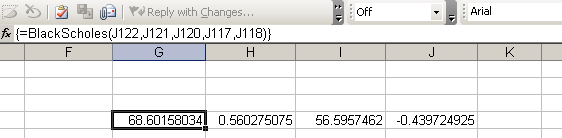Categories

## Excel Black-Scholes Function

[UPDATE: After reading this post, see here for an updated version of the spreadsheet].

The Black-Scholes option valuation formula for an option paying a continuous dividend yield is the following:WhereandAttached is a simple Excel function that calculates the Black-Scholes option value for a specific set of input parameters. Currently, it just calculates the call value – if you use it as an array function, it will return a 4-element array with call value, call delta, put value, put delta, respectively. You could extend it pretty easily to calculate the rest of the Greeks.

Here is the code for the function (To create an Excel function, press ALT-F11 in your workbook and select Insert>Module. Note that the dividend yield parameter is optional.

Function BlackScholes(SpotPrice As Double, ExercisePrice As Double,
TimeToMaturity As Double, RiskFreeRate As Double, sigma As Double,
Optional DividendYield As Double) As Double()

Dim d1 As Double
Dim d2 As Double
Dim Nd1 As Double
Dim Nd2 As Double
Dim ResultArray() As Double

ReDim ResultArray(4) As Double

If (IsMissing(DividendYield)) Then
d1 = WorksheetFunction.Ln(SpotPrice / ExercisePrice) +
((RiskFreeRate + (0.5 * (sigma ^ 2))) * TimeToMaturity)
Else
d1 = WorksheetFunction.Ln(SpotPrice / ExercisePrice) +
((RiskFreeRate - DividendYield + (0.5 * (sigma ^ 2))) * TimeToMaturity)
End If

d1 = d1 / (sigma * (TimeToMaturity ^ (1 / 2)))
d2 = d1 - (sigma * (TimeToMaturity ^ (1 / 2)))
Nd1 = WorksheetFunction.NormSDist(d1)
Nd2 = WorksheetFunction.NormSDist(d2)

'Call Value
If (IsMissing(DividendYield)) Then
ResultArray(0) = (SpotPrice * Nd1)
- (ExercisePrice * Exp(-RiskFreeRate * TimeToMaturity) * Nd2)
Else
ResultArray(0) = Exp(-DividendYield * TimeToMaturity) * (SpotPrice * Nd1)
- (ExercisePrice * Exp(-RiskFreeRate * TimeToMaturity) * Nd2)
End If

'Call Delta
ResultArray(1) = Nd1

'Put Value
If (IsMissing(DividendYield)) Then
ResultArray(2) = Exp(-RiskFreeRate * TimeToMaturity)
* ExercisePrice * (1 - Nd2) - SpotPrice * (1 - Nd1)
Else
ResultArray(2) = Exp(-RiskFreeRate * TimeToMaturity) * ExercisePrice
* WorksheetFunction.NormSDist(-d2) - Exp(-DividendYield * TimeToMaturity)
* SpotPrice * WorksheetFunction.NormSDist(-d1)
End If

'Put delta
ResultArray(3) = -WorksheetFunction.NormSDist(-d1)

BlackScholes = ResultArray
End Function


Save this, and set up the input parameters in Excel. Select a range of 4 cells, and then click the f(x) function selection button. Choose from the list of User Defined functions, and then select BlackScholes. Excel will prompt you for the input parameters:When you are finished inputing the parameters, press CTRL+SHIFT+ENTER to execute the function. Excel will populate the four cells with the calculated option values:A sample workbook is attached:BlackScholes.xls

Categories

## Data Precision, Excel, and Commons::Math

A post on the Jakarta User mailing list piqued my interest this week. The poster had noticed that they were getting significantly different results for some statistical measures from the output of Commons::Math vs. what Excel was producing. This, if true, would be a pretty serious situation. Excel’s calculation engine is proven and very mature (I know one of the guys who works on it, and he’s a genius), so any discrepancy would seem to point to Commons::Math.

Needless to say, the best way to verify this is with a simple “spike”, as the agile guys would say. So I fired up Excel, and using its random number generator, produced 20,000 normally distributed numbers with a standard deviation of 1,000 and a mean of 5,000. I used the  Tools > Data Analysis add-in to do this, but you could also use the =NORMINV(rand(),mean,standard_dev) function.

When this was complete, I exported the data to a text file, and read in the values and calculated some simple stats using Commons::Math. Here is the sample program, if you’re interested:

 package uk.co.researchkitchen.math; import java.io.BufferedReader; import java.io.FileReader; import java.io.IOException; import org.apache.commons.math.stat.descriptive.moment.Mean; import org.apache.commons.math.stat.descriptive.moment.StandardDeviation; import org.apache.commons.math.stat.descriptive.moment.Variance; import org.apache.commons.math.stat.descriptive.rank.Median; public class TestPrecision {      static final int DATA_SIZE = 20000;      double[] data = new double[DATA_SIZE];      public static void main(String[] args) throws IOException {     TestPrecision testPrecision = new TestPrecision();     // Book1.txt is an exported Excel spreadsheet containing     // 20,000 normally distributed numbers with a mean of ~5,000     // and a stdev (sigma) of ~1,000     testPrecision.calculateStats("c:\\temp\\Book1.txt");   }      public void calculateStats(String filename) throws IOException {     BufferedReader br = new BufferedReader(new FileReader(filename));          int count = 0;     String line = null;     while ((line = br.readLine()) != null) {       double datum = Double.valueOf(line);       System.out.println(datum);       data[count++] = datum;     }          System.out.println("Read " + count + " items of data.");          System.out.println("Standard deviation = " + new StandardDeviation().evaluate(data));     System.out.println("Median = " + new Median().evaluate(data));     System.out.println("Mean = " + new Mean().evaluate(data));     System.out.println("Variance = " + new Variance().evaluate(data));   } }

I then went back to Excel and calculated the same measures in there (calculating both the 1/(N-1) sample and 1/N population standard deviation and variance). Here are the tabulated results:

 Commons Math Excel Standard Deviation 1005.8672054459015 1005.8672054332 Median 50011.934275 50011.9342750000 Mean 50008.74172390576 50008.7417239057 Variance 1011768.8349915474 1011768.8349659700

As suspected, they are almost identical, bar some rounding differences, at least an order of magnitude closer than the figures in the post (I told Excel to limit the precision to 10 digits, hence some figures seem a smaller precision). I don’t know what precision Excel uses internally for these calculations. It may be interesting to write a BigDecimal-based equivalent of the statistics package in Commons::Math.

There may be other reasons why the numbers given in the example don’t match, but unless I’m missing something obscure, or specific to the use case shown, it looks like the issue is not with the internal implementation of [math] (which incidentally looks like a very very neat little toolkit).